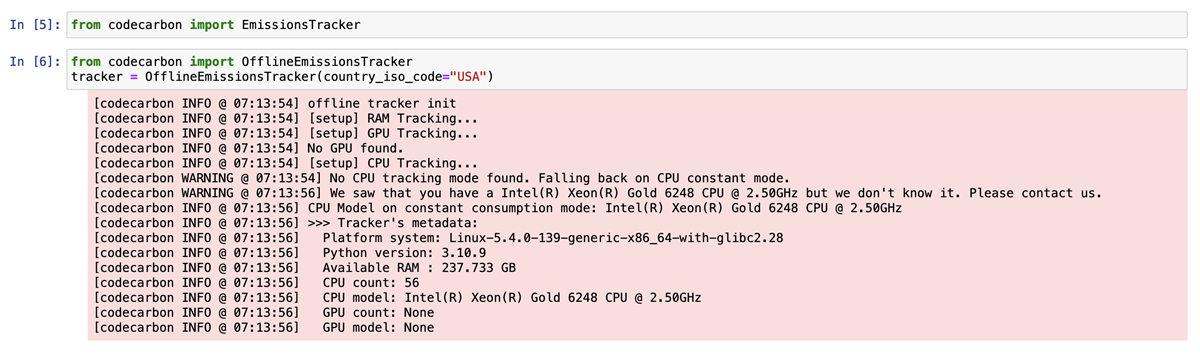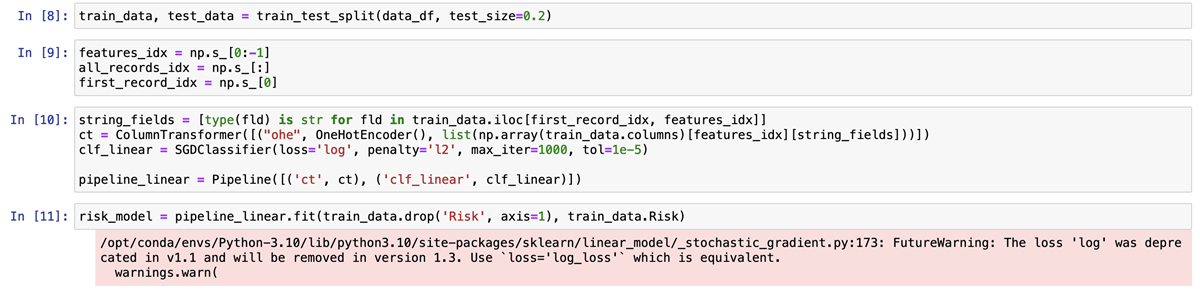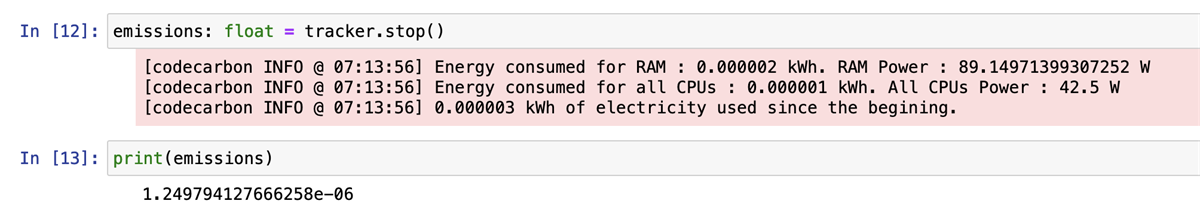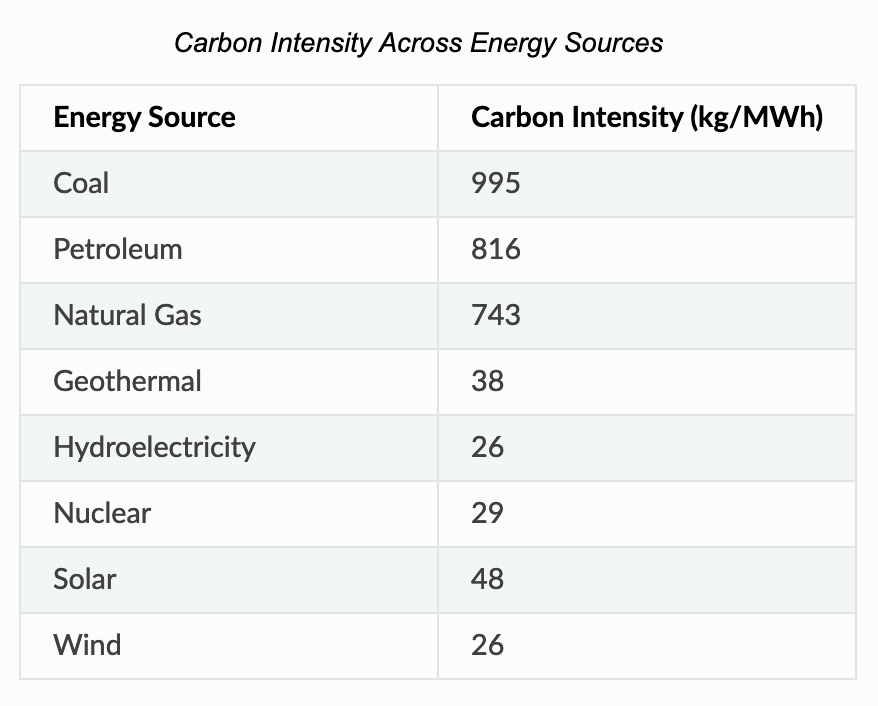# Global AI and Data Science

View Only

## How to Measure Carbon Emissions for Training ML Models

####By Rachana Vishwanathula posted Wed April 19, 2023 06:56 AM

Codecarbon  is a popular open-source python library for measuring carbon emissions. One of the things I liked about codecarbon is their dashboard. The carbon emissions estimation is done by measuring the power consumption of the total GPUs, CPUs, and RAM. Then it applies the regional carbon intensity of electricity of your cloud provider or country if you are using a local machine or on-premise cluster.

A sample ML model usually requires dataset upload -> splitting training/testing data -> train an ML model and evaluate it for accuracy. So what if I want to see carbon emissions while performing these tasks? How can I do it? The following lines of code will explain how to do that. I have uploaded the code here. I ran the code on Watson Studio but this can be calculated for any environment. I am using  german credit risk dataset to train a SGDC Classifier based ML model.

``````# import codecarbon library to calculate emissions
from codecarbon import EmissionsTracker
from codecarbon import OfflineEmissionsTracker

# initiate tracker
tracker = OfflineEmissionsTracker(country_iso_code="USA")
tracker.start()

# ML code
train_data, test_data = train_test_split(data_df, test_size=0.2)
features_idx = np.s_[0:-1]
all_records_idx = np.s_[:]
first_record_idx = np.s_
string_fields = [type(fld) is str for fld in train_data.iloc[first_record_idx, features_idx]]
ct = ColumnTransformer([("ohe", OneHotEncoder(), list(np.array(train_data.columns)[features_idx][string_fields]))])
clf_linear = SGDClassifier(loss='log', penalty='l2', max_iter=1000, tol=1e-5)
pipeline_linear = Pipeline([('ct', ct), ('clf_linear', clf_linear)])
risk_model = pipeline_linear.fit(train_data.drop('Risk', axis=1), train_data.Risk)

# stop the tracker and print emissions
emissions: float = tracker.stop()
print(emissions)``````

Once I ran the above code, here's the output.

1. Start tracker
A tracker is a function which should be initiated to start the tracking of emissions. All the code that is written after starting the tracker will be tracked for emissions. The following output shows when a tracker is initiated with the required parameters. This is done by initiating the EmissionsTracker method if it's online mode or OfflineEmissionsTracker method if it's offline (when not connected to internet) tracking. Offline mode can also be used if you want to give manual parameters to calculate carbon emissions using a specific region's data.2. Running ML model training
Once the tracker is started, run the ML model training. Once the training is done, stop the tracker.3. End Tracker and See Emissions
After running the ML model training, end the tracking and print the emissions.So, how is this calculated?
Carbon dioxide (CO₂) emissions, expressed as kilograms of CO₂-equivalents [CO₂eq], are the product of two main factors :
```C = Carbon intensity of the electricity consumed for computation: quantified as kg of CO₂ emitted per kilowatt-hour of electricity.

P = Power consumed by the computational infrastructure: quantified as kilowatt-hours.
```

Carbon dioxide emissions (CO₂eq) can then be calculated as `C * P`

i. Carbon Intensity

This toolkit calculates the Carbon Intensity of the electricity consumed based on the mix of fossil fuels and low-carbon energy sources in the local energy grid. When available, CodeCarbon uses global carbon intensity of electricity per cloud provider ( here ) or per country ( here ). If we don’t have the global carbon intensity or electricity of a country, and we have its electricity mix, we compute the carbon intensity of electricity using this table :for example, if the energy mix of the grid electricity is 25% coal, 35% petroleum, 26% natural gas and 14% nuclear:
`Net Carbon Intensity = 0.25 * 995 + 0.35 * 816 + 0.26 * 743 + 0.14 * 29 = 731.59 kgCO₂/kWh`
If the global carbon intensity of a country or it’s electricity mix is not available, then CodeCarbon applies a world average of 475 gCO2.eq/KWh

ii. Power Usage

Power supply to the underlying hardware is tracked at frequent time intervals with default value 15 seconds. Codecarbon is compatible with NVIDIA GPUs that support NVIDIA Management Library (NVML) and Intel CPUs that support Intel RAPL . If your CPU is not on the list of supported CPUs then it will estimate the power consumption of CPU as 50% of their thermal design power using default average 85W.
TDP (Thermal Design Power) for several supported infrastructure is listed here. The supported cloud providers are respective TDP is listed here. Further, you can initialize the tracker with a region. Region specific data is listed here.